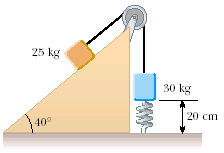# Why is it wrong when I try to use Newton's Law?

A 25 kg block is connected to a 30 kg block by a light string that passes over a frictionless pulley. The 30 kg block is connected to a light spring of force constant 195 N/m, as in Figure P13.59. The spring is unstretched when the system is as shown in the figure, and the incline is smooth. The 25 kg block is pulled 15 cm down the incline (so that the 30 kg block is 35 cm above the floor) and is released from rest. Find the speed of each block when the 30 kg block is 20 cm above the floor (that is, when the spring is unstretched).I wrote out all the equations

For the 25kg mass

$$\sum F_x = T- mg\sin\theta = ma$$

$$\sum F_y = n = mg\cos\theta$$

For the 30kg mass

$$\sum F_y = kx + Mg - T = Ma$$

Using energy, I got v = 0.91m/s, but using Newton's Law I got 0.95m/s

EDIT:

Energy

$E = Mgh + \frac{1}{2}kh^2$

$E' = mgh\sin\theta + \frac{(M + m)}{2}v^2$

$E = E'$

Solving, $v = \sqrt{\frac{2Mgh + kh^2 - mgh\sin\theta}{m + M}}$

Forces

$T- mg\sin\theta = ma$

$kh + Mg - T = Ma$

$kx + g(M - m\sin\theta) = (M + m)a$

$\frac{kh + g(M - m\sin\theta)}{M + m} = a$

$v^2 = 2\frac{kh + g(M - m\sin\theta)}{M + m}h$

$v = \sqrt{2\frac{kh + g(M - m\sin\theta)}{M + m}h}$

The source of the mistake seem to lie with the 2 in front of the kh

• Could you edit in the rest of your work using each method? – David Z Feb 12 '12 at 20:38
• It's not clear what $M$ and $m$ are. Could you label them $m_1$ and $m_2$ (if they are the mass of the first and second blocks respectively)? – Dan Feb 12 '12 at 21:58
• M is 30kg and m is 25kg. I thought big M would help to mean that the mass is larger. – Hawk Feb 12 '12 at 22:01
• I thought that was clear (though it never hurts to say it explicitly). You should explain the meanings of some of your other variables, though ($h$ in particular). – David Z Feb 12 '12 at 22:08
• Oh h is 0.15m. Sorry everyone – Hawk Feb 12 '12 at 22:15

You are using the following formula:

$$v^2 = 2 a x$$

which is only valid when the acceleration "a" is independent of x. You are applying this formula to the spring case, where the spring force is different at different x. This is not valid, because the acceleration produced by the spring is different at different positions. The correct formula generalizing the above to the case of non-constant acceleration is the conservation of energy formula.

For the case of a unit mass on a spring, if you stretch the spring by an amount X, you have an acceleration of kX, and multiplying by distance X, you find that the velocity squared would be

$$V^2 = 2 kX^2$$

This is exactly twice the correct answer, which is found by conservation of energy

$${V^2\over 2} = {kX^2\over 2}$$

This explains your unwanted factor of 2.

• Ron strikes again! – Timtam Feb 13 '12 at 3:18